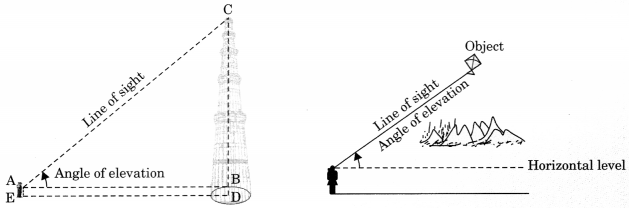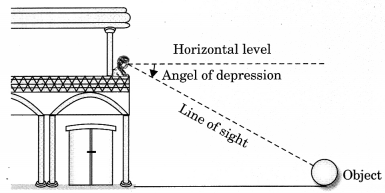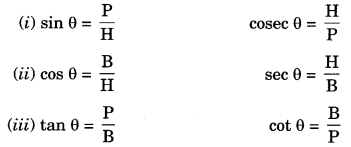On this page, you will find Some Applications of Trigonometry Class 10 Notes Maths Chapter 9 Pdf free download. CBSE NCERT Class 10 Maths Notes Chapter 9 Some Applications of Trigonometry will seemingly help them to revise the important concepts in less time.

## CBSE Class 10 Maths Chapter 9 Notes Some Applications of Trigonometry

### Some Applications of Trigonometry Class 10 Notes Understanding the Lesson

Trigonometry is the study of relationships between the sides and angles of a triangle. In this chapter you will study about some ways in which trigonometry is used:

• It is used in geography and in navigation.
• It is used in constructing maps, determine the position of an island in relation to the longitudes and latitudes.
• It is used for calculating the height and distance of various objects without measuring it.

Terms related to height and distance:

1. Line of sight: The line joining the eyes of the observer and the objects which he/she observes is called line of sight.2. Angle of elevation: (When object is above the horizontal)
The angle between the line of sight and the horizontal is called the angle of elevation.3. Angle of depression: (When object is below the horizontal)
The angle between the horizontal line and the line of sight is called the angle of depression.Trigonometric formulae used: